# Power and measuring itPage 3

#### WATCH ALL SLIDES

If a motor lifts the water

from a depth ‘h1’ and then

raises to a height ‘h2’ then

the power of the motor is

given by

9/3/2015

16

RGUIIIT BASAR

Slide 17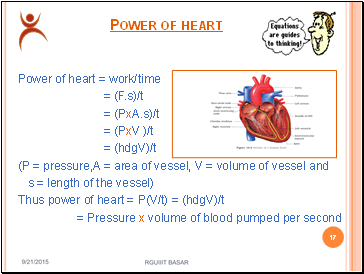## Power of heart

Power of heart = work/time

= (F.s)/t

= (PxA.s)/t

= (PxV )/t

= (hdgV)/t

(P = pressure,A = area of vessel, V = volume of vessel and

s = length of the vessel)

Thus power of heart = P(V/t) = (hdgV)/t

= Pressure x volume of blood pumped per second

9/3/2015

17

RGUIIIT BASAR

Slide 18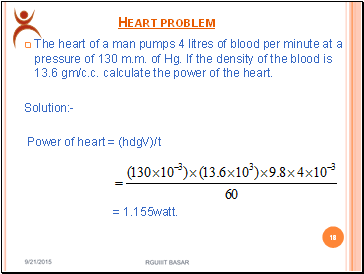## Heart problem

The heart of a man pumps 4 litres of blood per minute at a pressure of 130 m.m. of Hg. If the density of the blood is 13.6 gm/c.c. calculate the power of the heart.

Solution:-

Power of heart = (hdgV)/t

= 1.155watt.

9/3/2015

18

RGUIIIT BASAR

Slide 19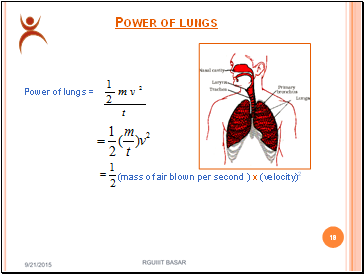## Power of lungs

Power of lungs =

(mass of air blown per second ) x (velocity)2

9/3/2015

19

RGUIIIT BASAR

Slide 20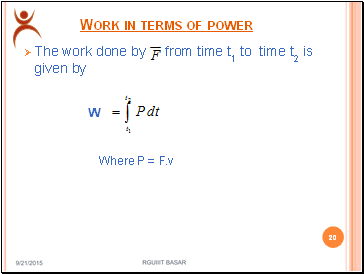## Work in terms of power

The work done by from time t1 to time t2 is given by

Where P = F.v

9/3/2015

20

RGUIIIT BASAR

W

Slide 21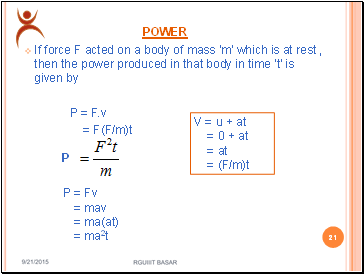## Power

If force F acted on a body of mass ‘m’ which is at rest , then the power produced in that body in time ‘t’ is given by

P = F.v

= F(F/m)t

9/3/2015

21

RGUIIIT BASAR

V = u + at

= 0 + at

= at

= (F/m)t

P

P = Fv

= mav

= ma(at)

= ma2t

Slide 22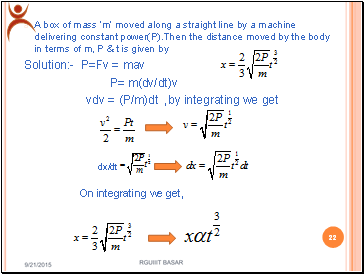A box of mass ‘m’ moved along a straight line by a machine delivering constant power(P).Then the distance moved by the body in terms of m, P & t is given by

Solution:- P=Fv = mav

P= m(dv/dt)v

vdv = (P/m)dt ,by integrating we get

dx/dt

On integrating we get,

9/3/2015

22

RGUIIIT BASAR

Slide 23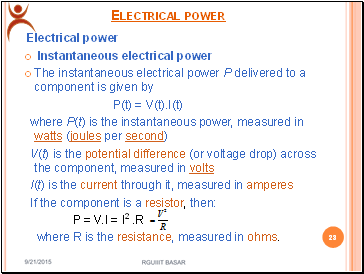## Electrical power

Electrical power

Instantaneous electrical power

The instantaneous electrical power P delivered to a component is given by

P(t) = V(t).I(t)

where P(t) is the instantaneous power, measured in watts (joules per second)

V(t) is the potential difference (or voltage drop) across the component, measured in volts

I(t) is the current through it, measured in amperes

If the component is a resistor, then:

P = V.I = I2 .R

where R is the resistance, measured in ohms.

9/3/2015

Go to page:
1  2  3  4  5